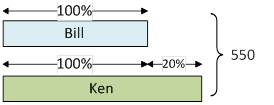### Sample Problem

Ken and Bill collected 550 stickers together. Ken collected 20% more stickers than Bill. How many stickers did Ken collect?

stickers

#### Solution

Consider Bill's stickers 100%, and Ken's stickers is (100% + 20% = 120%).

Then, (100 + 120) % times the number of Bill's stickers is 550.Use the formula: the amount of Sum ÷ the percentage of sum = smaller number

Bill's tickers: 550 ÷ (100 + 120) % = 550 ÷ 220% = 250

The bigger number = whole number – smaller number

Thus, Ken's stickers: 550 – 250 = 300 stickers